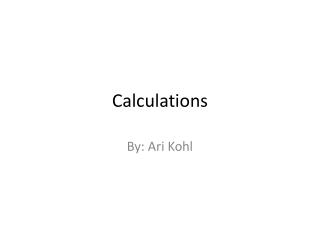DownloadDownload PresentationCalculations

# Calculations

Download Presentation## Calculations

- - - - - - - - - - - - - - - - - - - - - - - - - - - E N D - - - - - - - - - - - - - - - - - - - - - - - - - - -
##### Presentation Transcript

1. Calculations By: Ari Kohl

2. How to Calculate Area of a Circle • ∏ radius squared.

3. How to Calculate Circumference • ∏ diameter.

4. Exponential Notation 45 = 1,024 23 = 8

5. Jeopardy ½ T or F ½ Multiple Choice

6. 100 Points Area of a circle The radius is 7”. The diameter is 14”. What is the area? • 14” • 154” • 390” • 7,834”

7. 200 Points Area of a circle The radius is 5”. The circumference is 31.4”. What is the area? • 72” • 39” • 48” • 79”

8. 300 Points Area of a circle If the radius of this circle is 720” then the area is 2,160. T or F?

9. 400 Points Area of a circle The diameter is 7’ the radius is 3.5’. The area is 38. T or F?

10. 100 Points Circumference The radius is 5cm. What is the circumference? • 376cm • 34cm • 56cm • 31cm

11. 200 Circumference The diameter is 34”. The circumference is 123. T or F?

12. 300 Circumference The radius is 24”. What is the circumference? • 48” • 64” • 151” • 900”

13. 400 Circumference The diameter is 15. The circumference is 47. T or F?

14. 100 Points Exponential Notation Answer this yes or no question. 64 8^2 Y or N?

15. 200 Points Exponential Notation 5^6= • 30 • 72 • 90 • 15,625

16. 300 Points Exponential Notation Answer this yes or no question. 49 7^7 Y or N?

17. 400 Points Exponential Notation 7^8= • 56 • 78 • 92 • 5,764,801

18. Wrong!

19. Right!

20. Wrong!

21. Wrong!

22. Wrong!

23. Wrong!

24. Wrong!

25. Right!

26. Wrong!

27. Right!

28. Right!

29. Wrong!

30. Wrong!

31. Wrong!

32. Wrong!

33. Right!

34. Wrong!

35. Right!

36. Wrong!

37. Wrong!

38. Right!

39. Wrong!

40. Right!

41. Wrong!

42. Wrong!

43. Wrong!

44. Wrong!

45. Right!

46. Wrong!

47. Right!

48. Congratulations!